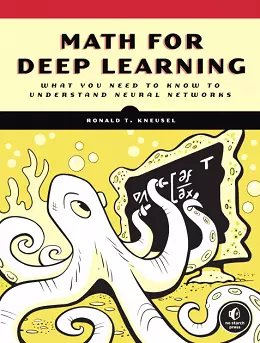# Math for Deep Learning### eBook Details:

• Paperback: 344 pages
• Publisher: WOW! eBook (November 23, 2021)
• Language: English
• ISBN-10: 1718501900
• ISBN-13: 978-1718501904

### eBook Description:

Math for Deep Learning: What You Need to Know to Understand Neural Networks provides the essential math you need to understand deep learning discussions, explore more complex implementations, and better use the deep learning toolkits

Deep learning is everywhere, making this powerful driver of AI something more STEM professionals need to know. Learning which library commands to use is one thing, but to truly understand the discipline, you need to grasp the mathematical concepts that make it tick. This Math for Deep Learning book will give you a working knowledge of topics in probability, statistics, linear algebra, and differential calculus – the essential math needed to make deep learning comprehensible, which is key to practicing it successfully.

Each of the four subfields are contextualized with Python code and hands-on, real-world examples that bridge the gap between pure mathematics and its applications in deep learning. Chapters build upon one another, with foundational topics such as Bayes’ theorem followed by more advanced concepts, like training neural networks using vectors, matrices, and derivatives of functions. You’ll ultimately put all this math to use as you explore and implement deep learning algorithms, including backpropagation and gradient descent – the foundational algorithms that have enabled the AI revolution.

You’ll learn:

• The rules of probability, probability distributions, and Bayesian probability
• The use of statistics for understanding datasets and evaluating models
• How to manipulate vectors and matrices, and use both to move data through a neural network
• How to use linear algebra to implement principal component analysis and singular value decomposition